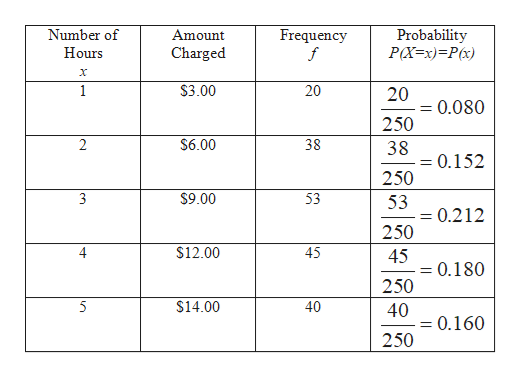# The Downtown Parking Authority of Tampa, Florida, reported the following information for a sample of 250 customers on the number of hours cars are parked and the amount they are charged. Number of Hours Frequency Amount Charged1 20 \$3.002 38  6.003 53  9.004 45  12.005 40  14.006 13  16.007 5  18.008 36  20.00  250     Click here for the Excel Data File a-1. Convert the information on the number of hours parked to a probability distribution. (Round your answers to 3 decimal places.)a-2. Is this a discrete or a continuous probability distribution?b-1. Find the mean and the standard deviation of the number of hours parked.b-2. How long is a typical customer parked? (Round your answer to 3 decimal places.)Find the mean and the standard deviation of the amount charged. (Round your answers to 2 decimal places.)

Question
88 views

The Downtown Parking Authority of Tampa, Florida, reported the following information for a sample of 250 customers on the number of hours cars are parked and the amount they are charged.

 Number of Hours Frequency Amount Charged 1 20 \$ 3.00 2 38 6.00 3 53 9.00 4 45 12.00 5 40 14.00 6 13 16.00 7 5 18.00 8 36 20.00 250

a-1. Convert the information on the number of hours parked to a probability distribution. (Round your answers to 3 decimal places.)

a-2. Is this a discrete or a continuous probability distribution?

b-1. Find the mean and the standard deviation of the number of hours parked.

b-2. How long is a typical customer parked? (Round your answer to 3 decimal places.)

Find the mean and the standard deviation of the amount charged. (Round your answers to 2 decimal places.)

check_circle

Step 1

Given information:

Sample size (n) = 250

The number of hours cars are parked and the amount they are charged is:

Table 1

 Number of Hours Frequency Amount Charged 1 20 \$3.00 2 38 \$6.00 3 53 \$9.00 4 45 \$12.00 5 40 \$14.00 6 13 \$16.00 7 5 \$18.00 8 36 \$20.00 Total 250
Step 2

a-1

Explanation:

Let x is a random variable of hours car are parked.

The probability formula for each frequency is:

Step 3

Here, N is population size and f  is frequency.

Convert the information on the number of hou...help_outlineImage TranscriptioncloseProbability P(Xx)=Px) Number of Amount Frequency f Charged Hours х 20 \$3.00 1 20 = 0.080 250 2 \$6.00 38 38 = 0.152 250 \$9.00 3 53 53 = 0.212 250 \$12.00 4 45 45 = 0.180 250 \$14.00 40 40 = 0.160 250 fullscreen

### Want to see the full answer?

See Solution

#### Want to see this answer and more?

Solutions are written by subject experts who are available 24/7. Questions are typically answered within 1 hour.*

See Solution
*Response times may vary by subject and question.
Tagged in

### Statistics